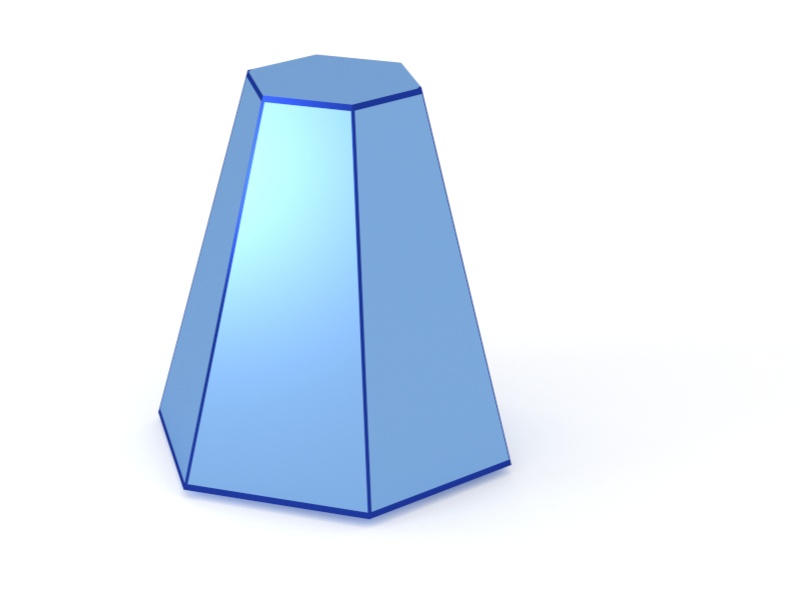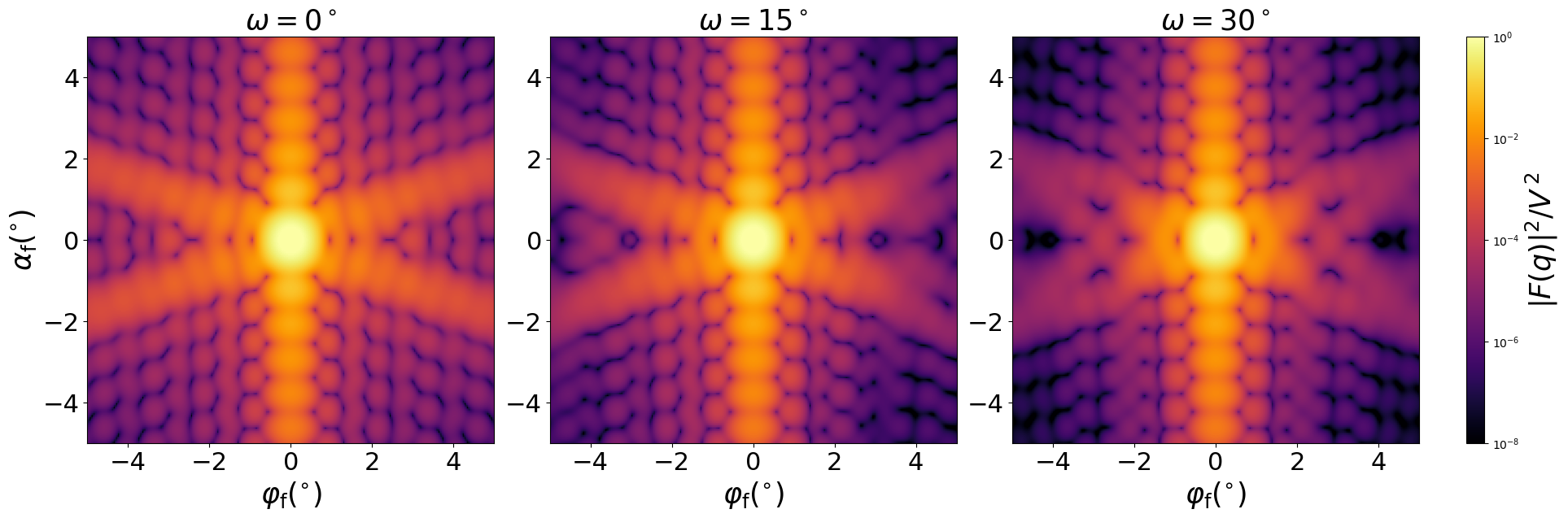### Pyramid6

A truncated pyramid (frustum) based on a regular hexagon.#### Constructor

Pyramid6(R, H, alpha)


Parameters:

• R, edge of the regular hexagonal base
• H, height
• alpha, dihedral angle $\alpha$ between the base and a side face.

Constraint:

$H \le \dfrac{\sqrt3\tan\alpha}{2} R$

Note that the orthographic projection does not show $\alpha$ but the angle $\beta$ between base and a side edge. They are related through $\sqrt3\tan\alpha = 2\tan\beta$.

#### Usage

As for any other Form factor.

#### Implementation

Class Pyramid6 inherits from the interface class IFormFactor .

Form factor computation is based on the generic form factor of a polyhedron provided by libformfactor .

Volume has been validated against $$V=\dfrac{3}{4}R^3\tan\alpha\Big[1-\Big(1-\dfrac{2H}{\sqrt3R\tan\alpha}\Big)^3\Big].$$

More special:

• Prism6, if $\alpha=90^{\circ}$.

#### Example

Scattering by uncorrelated, oriented pyramids for horizontal incidence. Rotation around $z$ axis:Generated by Examples/ff/Pyramid6.py .

#### History

Was named “Cone6” until BornAgain 1.19.

Corresponds to “Cone6” in IsGISAXS [manual, Eq. 2.31] and “Cone with six fold symmetry” [Renaud 2009, Eq. 222]. In BornAgain 1.6, redefined to let the $x$ axis lie in a mirror plane (rotated by $30^{\circ}$ with respect to the previous version).

Up to BornAgain 1.5 by numerical integration, as in IsGISAXS. Since BornAgain 1.6 higher speed and better accuracy are achieved by using the generic form factor of a polyhedron [Wuttke 2021] with series expansions near singularities.